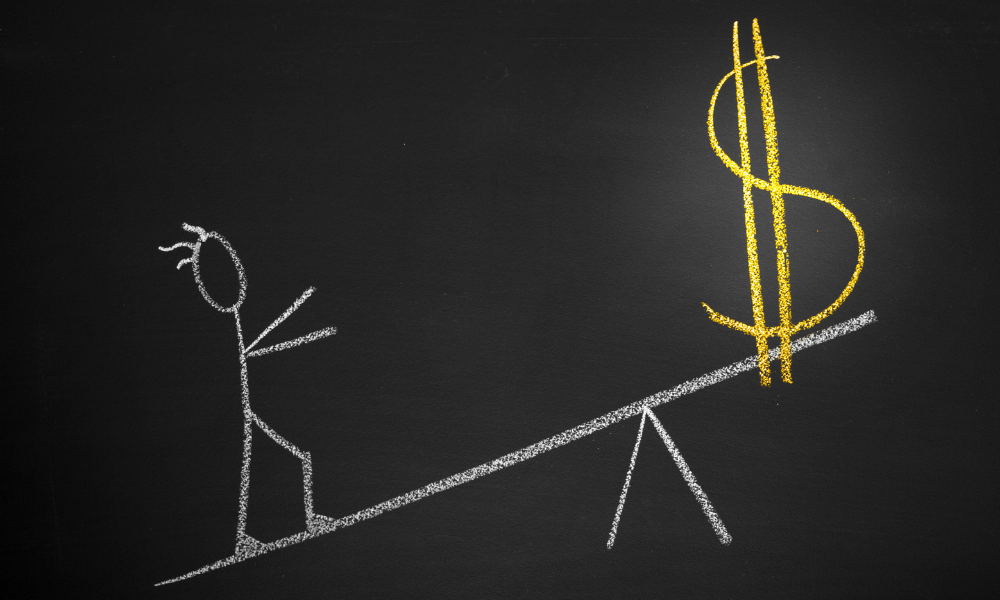## Forex Lot Size vs. Leverage - ForexProp

The terms used by participants in the Forex market can be confusing for novice traders. But everyone who comes to the exchange to earn money should understand these concepts. Below we will look at such key concepts as leverage and lot size on Forex, and find out what pips are.Leverage vs lot size are different concepts on Forex, but there is a certain connection between them. Let's figure out what are leverage and lots means.

Leverage means that the trader borrows funds from their Forex broker or a related third party. With this financial support, they can open trades more effectively than without leverage.

Now let's define the concept of lot on Forex.

The trader sets the volume in contracts when opening a position. Its value can be from 0.01 to 100.

It is important for beginners on Forex to remember the connection between the concepts of forex lot size and leverage.

Leverage actually doesn’t affect the size of the contract and its price. However, the concept of leverage plays a significant role in determining the size of a trader's position. The greater the leverage, the more a trader can afford to buy or sell large lots in quantities that are many times greater than their own funds.

## What are the pips?

Above we have discussed what lot and leverage are. The connecting link between these two concepts is a pip (short for percentage in point). It represents the minimum fraction of the change in the value of a trading instrument.

In other words, a pip is the standard smallest unit of measure by which a currency quote can change. On the foreign exchange market, 1 pip is usually equal to \$0.00001 in pairs with the US dollar.

Oil and stocks, for example, have two characters after the decimal point. So the last (second after the decimal point) figure is a pip for these assets.

Let's look at the concept of a pip through an example. This way we can clearly see the relationship between lot size and leverage on Forex. Suppose we have a direct quote of EURUSD at 1.18699. This means that 1 Euro is worth 1.18699 USD.

If this quotation grew by one point (up to 1.18700), the value of 1 US dollar would decrease relative to the euro, since now you have to pay 0.00001 USD more for 1 euro.

Even 1 pip of price change has a direct impact on the final value of the trade.

The standard size of one contract for most brokers is 100,000 units. 1 unit of EURUSD will be equal to 1.18699 USD.

## Example:

Suppose an investor buys 0.1 lots, hence the contract size will be \$11,869.9 (100,000 * 0.1 * 1.18699). Suppose the exchange rate of this pair increases by one pip. Then the price of the contract of the same size will be equal to \$11,870.0.

So the cost of 1 pip with a 0.1 contract will be equal to 0.1 USD.

An investor can buy much more with leverage. Suppose that our trader uses a 1:100 leverage and can increase the position by 100 times – they will not buy 0.1, but 10 lots. With such a large position, the cost of 1 pip will be 10 USD.

This example clearly shows how leverage affects the value of a pip through trade size. The more leverage, the larger position a trader can open. The larger the position, the higher the value of one point. Continue reading with Litefinance.com...